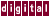## 6.5.2 IPA Example

In the following example, the variables `n` and `np1` have a simple relationship. This relationship is hidden behind a function call, however, so KAP normally does not try to concurrentize the loop in the main program. When the `/ipa=rxgfs` command qualifier is specified, KAP inspects the named function for information on the relationship of its arguments and returned value and the surrounding code. The assumed dependence is lifted and the loop can be safely concurrentized. If a function cannot be inlined, or if you do not want to inline it, it can often still be analyzed for its effects on the calling function.

The following example was run with the default values for ``` /optimize``` and `/scalaropt` .

```main()
{
int np1, i, m, n;
int a;

np1 = rxgfs( n );
for ( i=0; i<m; i++ ) {
a[i][n] = a[i-1][np1];
}

}

int rxgfs( n )
int n;
{
return (n+1);
}
```

Becomes:

```int main(  )
{
int np1;
int i;
int m;
int n;
int a;

np1 = rxgfs ( n ) ;
{
for ( i = 0; i<m; i++ ) {
a[i][n] = a[i-1][np1];
}
}
}
```

The subfunction was not shown.

Previous Page Next Page Contents Index
Command-Line Qualifiers